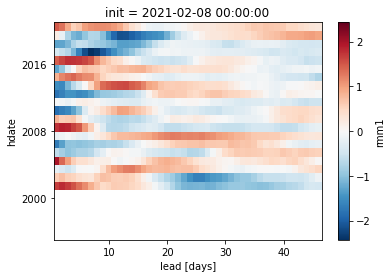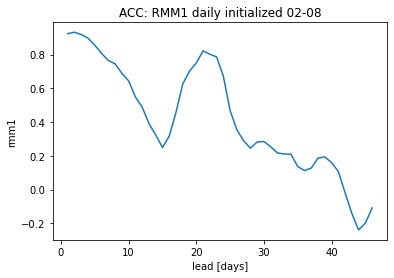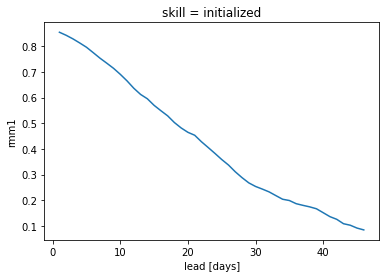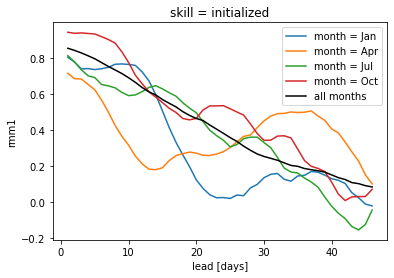# Calculate skill of a MJO Index of S2S models as function of daily lead time#

```# linting
```
```import xarray as xr

xr.set_options(display_style="html")

import numpy as np
import matplotlib.pyplot as plt
from climpred import HindcastEnsemble
import climpred
```
```Warning: ecCodes 2.21.0 or higher is recommended. You are running version 2.20.0
```

IRIDL hosts various subseasonal initialized forecast and hindcast simulations:

• `S2S project`:

• http://iridl.ldeo.columbia.edu/SOURCES/.ECMWF/.S2S/

• hindcast/reforecast: one variable, one model: ~ 80 GB

• `SubX project`:

• http://iridl.ldeo.columbia.edu/SOURCES/.Models/.SubX/

• hindcast/reforecast: one variable, one model: ~ 100 GB

Here, we demonstrate how to set a cookie for IRIDL and access the skill of RMM1 subseasonal reforecasts.

Here are instructions for configuring xarray to open protected Data Library datasets, after you have created a Data Library account and accepted the terms and conditions for the dataset.

1. Visit https://iridl.ldeo.columbia.edu/auth/genkey . Log in to the Data Library. Copy the key from the response.

2. Create a file with the following content, substituting the key from step 1 for `"xxxx"`: `Set-Cookie: __dlauth_id=xxxx; domain=.iridl.ldeo.columbia.edu`

3. Put the following in `~/.daprc`, which is `/home/jovyan/.daprc` on renku, substituting the path to the above file for `/path/to/cookie/file`: `HTTP.COOKIEJAR=/path/to/cookie/file`. You may need to copy `.daprc` to `/home/jovyan` on renku, because `/home/jovyan` is not tracked by `git`.

```!cat ~/.daprc
```
```HTTP.COOKIEJAR=/Users/aaron.spring/.cookie_iridl
```
```#%writefile ~/.cookie_iridl
```

## Get observations#

```# pre-computed
"rmm1"
].to_dataset()  # only until 2017
obsds = obsds.dropna("time").sel(time=slice("1995", None))  # Get rid of missing times.
```

## Get `on-the-fly` reforecasts#

S2S models:

• `ECMF`

• `ECCC`

• `HMCR`

• `KMA`

• `UKMO`

There are a set of reforecasts of the ECMWF model that match each real time forecast. They are made “on the fly” when a real time forecast is issued. So for S=0000 8 Feb 2021, there are reforecasts initialized on 0000 8 Feb 2020 and the 19 previous years on 8 Feb.

```%%time
fcstds = xr.open_dataset(
"https://iridl.ldeo.columbia.edu/SOURCES/.ECMWF/.S2S/.ECMF/.reforecast/.RMMS/.ensembles/.RMM1/dods",
decode_times=False,
chunks=None,
).compute()
```
```CPU times: user 613 ms, sys: 1.06 s, total: 1.67 s
Wall time: 24.6 s
```
```# calendar '360' not recognized, but '360_day'
if fcstds.hdate.attrs["calendar"] == "360":
fcstds.hdate.attrs["calendar"] = "360_day"
```

The S2S data dimensions correspond to the following `climpred` dimension definitions: `M=member`, `S=init`. We will rename the dimensions to their `climpred` names.

```# rename to match climpred dims: https://climpred.readthedocs.io/en/stable/setting-up-data.html
fcstds = fcstds.rename({"S": "init", "L": "lead", "M": "member", "RMM1": "rmm1"})
```
```fcstds = xr.decode_cf(fcstds, use_cftime=True)
fcstds.coords
```
```Coordinates:
* hdate    (hdate) object 1995-07-01 00:00:00 ... 2020-07-01 00:00:00
* init     (init) object 2015-05-14 00:00:00 ... 2021-02-15 00:00:00
* member   (member) float32 0.0 1.0 2.0 3.0 4.0 5.0 6.0 7.0 8.0 9.0 10.0
* lead     (lead) timedelta64[ns] 1 days 2 days 3 days ... 45 days 46 days
```

### Skill for a single real-time forecast from corresponding reforecasts#

```# assessing the skill of the reforecasts done annually from 8 Feb 2001 to 8 Feb 2020
# for the real-time forecast 8 Feb 2021
d = "08"
m = "02"
y = "2021"

fcstds.sel(init=f"{y}-{m}-{d}").squeeze().rmm1.mean("member").plot()
```
```<matplotlib.collections.QuadMesh at 0x7ff84ebf9d90>
``````import cftime

# create a new init coordinate
new_init = xr.concat(
[
xr.DataArray(
cftime.DatetimeProlepticGregorian(int(h.dt.year.values), int(m), int(d))
)
for h in fcstds.hdate
],
"init",
)

# select new inits for same dayofyear, drop all NaNs
fcstds_date = (
fcstds.sel(init=f"{y}-{m}-{d}", drop=True)
.squeeze(drop=True)
.assign_coords(hdate=new_init)
.rename({"hdate": "init"})
.dropna("init", how="all")
)
```
```hindcast = HindcastEnsemble(fcstds_date)
```
```%time skill = hindcast.verify(metric='acc', comparison='e2o', dim='init', alignment='maximize')
```
```CPU times: user 1.8 s, sys: 24.5 ms, total: 1.82 s
Wall time: 1.99 s
```
```skill.rmm1.plot()
plt.title(f"ACC: RMM1 daily initialized {m}-{d}")
```
```Text(0.5, 1.0, 'ACC: RMM1 daily initialized 02-08')
```### skill over many initializations#

create large `xr.DataArray` with all `hdate` stacked into `init`

```# restricting myself to years 2001-2003 for faster computation
fcstds = fcstds.sel(hdate=slice("2001", "2003"))
obs_ds = obsds.sel(time=slice("2001", "2004"))
```
```%%time
fcstds_dates = []
# loop over all inits, ignoring leap day
for s in fcstds.init:
d = str(s.init.dt.day.values).zfill(2)
m = str(s.init.dt.month.values).zfill(2)
y = s.init.dt.year.values
if d == "29" and m == "02":
continue
new_init = xr.concat(
[
xr.DataArray(
cftime.DatetimeProlepticGregorian(int(h.dt.year.values), int(m), int(d))
)
for h in fcstds.hdate
],
"init",
)
# select new inits for same dayofyear, drop all NaNs
fcstds_date = (
fcstds.sel(init=f"{y}-{m}-{d}", drop=True)
.squeeze(drop=True)
.assign_coords(hdate=new_init)
.rename({"hdate": "init"})
.dropna("init", how="all")
)
if fcstds_date.init.size > 0:  # not empty
fcstds_dates.append(fcstds_date)

fcstds_dates = xr.concat(fcstds_dates, "init")

fcstds_dates = fcstds_dates.sortby(fcstds_dates.init)
```
```CPU times: user 17.4 s, sys: 243 ms, total: 17.7 s
Wall time: 19.7 s
```
```hindcast = HindcastEnsemble(fcstds_dates)
```
```%time skill_all = hindcast.verify(metric='acc', comparison='e2o', dim='init', alignment='maximize')

skill_all.rmm1.plot()
```
```CPU times: user 55.2 s, sys: 576 ms, total: 55.7 s
Wall time: 1min 1s
```
```[<matplotlib.lines.Line2D at 0x7ff81c3af460>]
```### skill when initialized in different months#

```import warnings

warnings.filterwarnings(
"ignore"
)  # ignore climpred UserWarnings triggered by verification.sel(init)
```
```%%time
for m in np.arange(1, 13, 3):
hindcast_month = hindcast.sel(init=fcstds_dates.init.dt.month == m)
month_name = hindcast_month.get_initialized().init[:2].to_index().strftime("%b")
skill = (
hindcast_month.verify(metric="acc", comparison="e2o", dim="init", alignment="maximize")
)
skill.rmm1.plot(label=f"month = {month_name}")
skill_all.rmm1.plot(label='all months',c='k')
plt.legend()
```
```CPU times: user 11.1 s, sys: 124 ms, total: 11.2 s
Wall time: 12.4 s
```
```<matplotlib.legend.Legend at 0x7ff81beeb190>
```## Get reforecasts without `on-the-fly`#

very similar workflow as in the `SubX` examples as there is no `hdate` coordinate: subseasonal SubX examples

S2S models:

• `CRNM`

• `CMA`

• `BOM`

• `ISAC`

• `JMA`

• `NCEP`

```%%time
fcstds = xr.open_dataset(
"https://iridl.ldeo.columbia.edu/SOURCES/.ECMWF/.S2S/.CNRM/.reforecast/.RMMS/.ensembles/.RMM1/dods",
decode_times=True,
).compute()

fcstds = fcstds.dropna("S", how="all")
```
```CPU times: user 454 ms, sys: 804 ms, total: 1.26 s
Wall time: 21.2 s
```
```# rename to match climpred dims: https://climpred.readthedocs.io/en/stable/setting-up-data.html
fcstds = fcstds.rename({"S": "init", "L": "lead", "M": "member", "RMM1": "rmm1"})
```
```hindcast = HindcastEnsemble(fcstds)
```%time skill = hindcast.verify(metric='acc', comparison='e2o', dim='init', alignment='maximize')
```CPU times: user 54.3 s, sys: 688 ms, total: 55 s
```skill.rmm1.plot()
```[<matplotlib.lines.Line2D at 0x7ff81bdf5be0>]